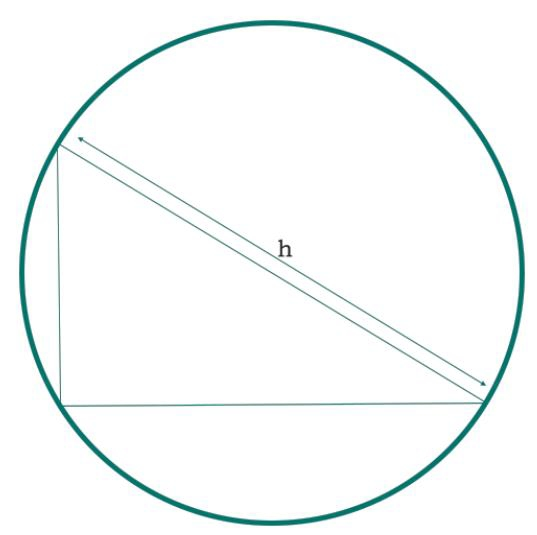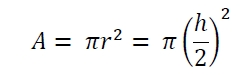# Area of Circumcircle of a Right Angled Triangle in C Program?

Here we will see how to get the area of circumcircle of a right angled triangle. The hypotenuse of the triangle is forming the diameter of the circle. So if the hypotenuse is h, then radius is h/2So the area is −## Example Code

#include <iostream>
#include <cmath>
using namespace std;
float area(float h) {
if (h < 0) //if h is negative it is invalid
return -1;
float area = 3.1415 * (h/2) * (h/2);
return area;
}
int main() {
float h = 8;
cout << "Area : " << area(h);
}

## Output

Area : 50.264

Updated on: 20-Aug-2019

88 Views